# NCERT Solutions for Class 12 Maths Chapter 12 Linear Programming

The NCERT Solutions for Class 12 Maths Chapter 12 Linear Programming is the most important study material to get good marks in the exams as well as to improve your knowledge of linear programming concepts. We prepare all the solutions of ch 12 linear programming in a conceptual way along with step by step explanations from subject experts. You can therefore grasp the concepts easily and have a good grasp of them before the exams.

These NCERT Solutions for Class 12 Maths Chapter 12 Linear programming can be used by students of UP, MP, Bihar, Gujarat, Uttarakhand, BIE, CBSE for a better understanding of concepts. You can score high in the board exams if you practice the step-by-step NCERT solutions. Please download the Important Questions, Notes, Exemplar Problems, Formulae, Study Materials from here & prepare to the best of your ability.

## Linear Programming Class 12 Ex 12.1

Ex 12.1 Class 12 Maths Question 1.
Maximize Z = 3x + 4y
subject to the constraints:
x + y ≤ 4,x ≥ 0,y ≥ 0.
Solution:
As x ≥ 0, y ≥ 0, therefore we shall shade the other inequalities in the first quadrant only. Now consider x + y ≤ 4.
Let x + y = 4 =>$frac { x }{ 4 } +frac { y }{ 4 } =1$
Thus the line has 4 and 4 as intercepts along the axes. Now (0, 0) satisfies the inequation i.e., 0 + 0 ≤ 4. Now shaded region OAB is the feasible solution.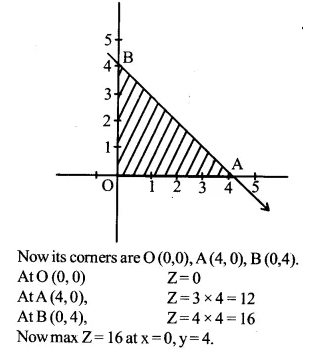Ex 12.1 Class 12 Maths Question 2.
Minimize Z = -3x+4y
subject to x + 2y ≤ 8,3x + 2y ≤ 12, x ≥ 0, y ≥ 0
Solution:
Objective function Z = -3x + 4y
constraints are x+2y ≤ 8,
3x + 2y ≤ 12, x ≥ 0,y ≥ 0
(i) Consider the line x+2y = 8. It pass through A (8,0) and B (0,4), putting x = 0, y = 0 in x + 2y ≤ 8,0 ≤ 8 which is true.
=> region x + 2y ≤ 8 lies on and below AB.Ex 12.1 Class 12 Maths Question 3.
Maximize Z = 5x+3y
subject to 3x + 5y ≤ 15,5x + 2y ≤ 10, x≥0, y≥0
Solution:
The objective function is Z = 5x + 3y constraints
are 3x + 5y≤15, 5x + 2y≤10,x≥0,y≥0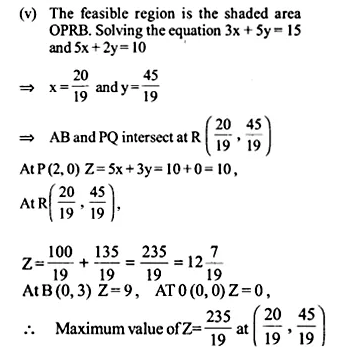Ex 12.1 Class 12 Maths Question 4.
Minimize Z = 3x + 5y such that x + 3y ≥ 3, x + y ≥ 2,x,y ≥ 0.
Solution:
For plotting the graph of x + 3y = 3, we have the following table: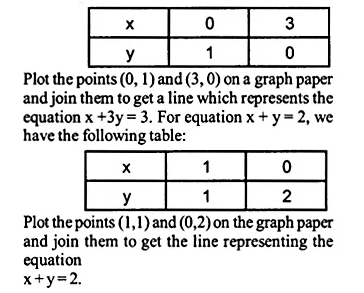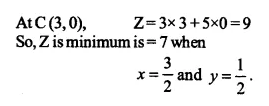Ex 12.1 Class 12 Maths Question 5.
Maximize Z=3x+2y subject to x+2y ≤ 10, 3x+y ≤ 15, x, y ≥ 0.
Solution:
Consider x + 2y ≤ 10
Let x + 2y = 10
=>$frac { x }{ 10 } +frac { y }{ 5 } =1$
Now (0,0) satisfies the inequation, therefore the half plane containing (0,0) is the required plane.
Again 3x+2y ≤ 15
Let 3x + y = 15
=>$frac { x }{ 5 } +frac { y }{ 15 } =1$
It is also satisfies by (0,0) and its required half plane contains (0,0).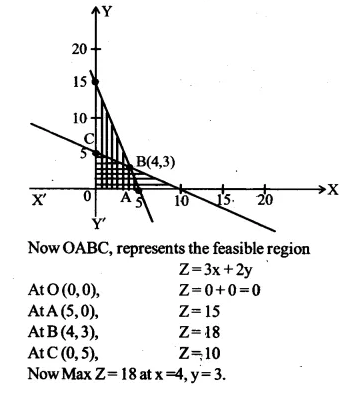Ex 12.1 Class 12 Maths Question 6.
Minimize Z = x + 2y subject to 2x + y ≥ 3, x + 2y ≥ 6, x, y ≥ 0.
Solution:
Consider 2x + y ≥ 3
Let 2x + y = 3
⇒ y = 3 – 2x(0,0) is not contained in the required half plane as (0, 0) does not satisfy the inequation 2x + y ≥ 3.
Again consider x+2y≥6
Let x + 2y = 6
=>$frac { x }{ 6 } +frac { y }{ 3 } =1$
Here also (0,0) does not contain the required half plane. The double-shaded region XABY’ is the solution set. Its comers are A (6,0) and B (0,3). At A, Z = 6 + 0 = 6
At B, Z = 0 + 2 × 3 = 6
We see that at both points the value of Z = 6 which is minimum. In fact at every point on the line AB makes Z=6 which is also minimum.

Show that the minimum of z occurs at more than two points.

Ex 12.1 Class 12 Maths Question 7.
Minimise and Maximise Z = 5x + 10y
subject to x + 2y ≤ 120, x + y ≥ 60, x – 2y ≥ 0, x,y≥0
Solution:
The objective function is Z = 5x + 10y constraints are x + 2y≤120,x+y≥60, x-2y≥0, x,y≥0Ex 12.1 Class 12 Maths Question 8.
Minimize and maximize Z = x + 2y subject to x + 2y ≥ 100,2x – y ≤ 0,2x + y ≤ 200;x,y ≥ 0.
Solution:
Consider x + 2y ≥ 100
Let x + 2y = 100
=>$frac { x }{ 100 } +frac { y }{ 50 } =1$
Now x + 2y ≥ 100 represents which does not include (0,0) as it does not made it true.
Again consider 2x – y ≤ 0
Let 2x – y = 0 or y = 2x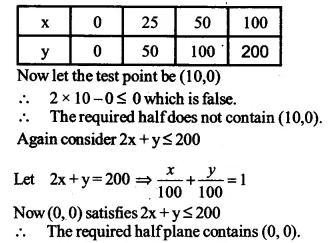Ex 12.1 Class 12 Maths Question 9.
Maximize Z = -x + 2y, subject to the constraints: x≥3, x + y ≥ 5, x + 2y ≥ 6,y ≥ 0
Solution:
The objective function is Z = – x + 2y.
The constraints are x ≥ 3, x + y ≥ 5, x + 2y ≥ 6, y ≥ 0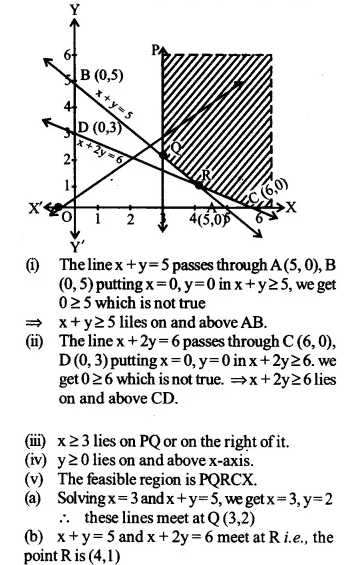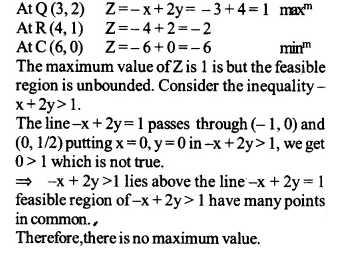Ex 12.1 Class 12 Maths Question 10.
Maximize Z = x + y subject to x – y≤ -1, -x + y≤0,x,y≥0
Solution:
Objective function Z = x + y, constraints x – y≤ -1, -x + y≤0,x,y≥0## Linear Programming Class 12 Ex 12.2

Ex 12.2 Class 12 Maths Question 1.
Reshma wishes to mix two types of food P and Q in such a way that the vitamin contents of the mixture contain at least 8 units of vitamin A and 11 units of vitamin B. Food P costs Rs 60/kg and Food Q costs Rs 80/kg. Food P contains 3 units/ kg of Vitamin A and 5 units/ kg of Vitamin B while food Q contains 4 units/kg of Vitamin A and 2 units/kg of vitamin B. Determine the minimum cost of the mixture.
Solution:
Let x kg of food P and y kg of food Q are mixed,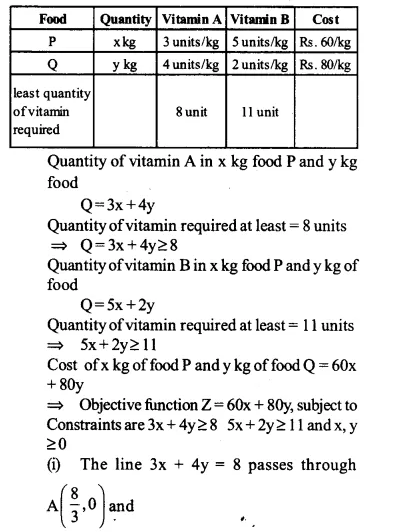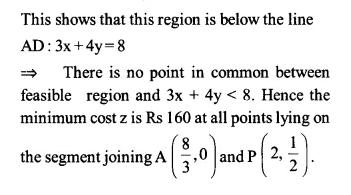Ex 12.2 Class 12 Maths Question 2.
One kind of cake requires 200 g of flour and 25g of fat, and another kind of cake requires 100 g of flour and 50 g of fat Find the maximum number of cakes which can be made from 5 kg of flour and 1 kg of fat assuming that there is no shortage of the other ingredients, used in making the cakes.
Solution:
Let number of cakes made of first kind are x and that of second kind is y.
∴ maximize Z = x + yEx 12.2 Class 12 Maths Question 3.
A factory makes tennis rackets and cricket bats. A tennis racket takes 1.5 hours of machine time and 3 hours of craftman’s time in its making while a cricket bat takes 3 hour of machine time and 1 hour of craftman’s time. In a day, the factory has the availability of not more than 42 hours of machine time and 24 hours of craftsman’s time.
(i) What number of rackets and bats must be made if the factory is to work at full capacity?
(ii) If the profit on a racket and on a bat is Rs 20 and Rs 10 respectively, find the maximum profit of the factory when it works at full capacity. .
Solution:
Let x tennis rackets and y cricket bats are produced in one day in the factory.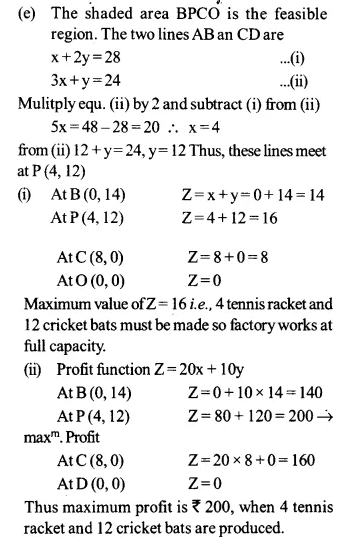Ex 12.2 Class 12 Maths Question 4.
A manufacturer produces nuts and bolts. It takes 1 hour of work on machine A and 3 hours on machine B to produce a package of nuts. It takes 3 hours on machine A and 1 hour on machine B to produce a package of bolts. He earns a profit of Rs 17.50 per package on nuts and Rs 7.00 per package on bolts. How many packages of each should be produced each day so as to maximise his profit, if he operates his machines for at the most 12 hours
Solution:
Let x nuts and y bolts are produced.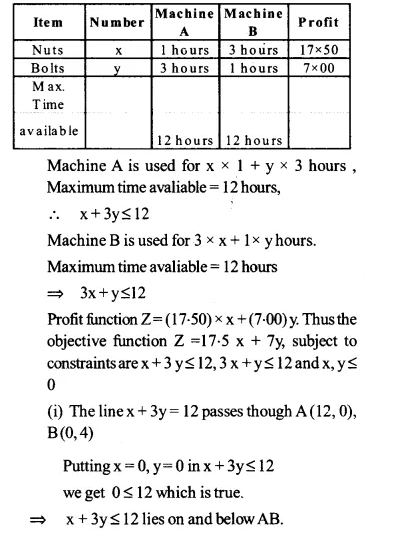Ex 12.2 Class 12 Maths Question 5.
A factory manufactures two types of screws, A and B, Each type of screw requires the use of two machines, an automatic and a hand operated. It takes 4 minutes on the automatic and 6 minutes on hand operated machines to manufacture a package of screws A, while it takes 6 minutes on automatic and 3 minutes on the hand operated machines to manufacture a package of screws B. Each machine is available for at the most 4 hours on any day. The manufacturer can sell a package of screws A at a profit of Rs 7 and screws B at a profit of Rs 10. Assuming that he can sell all the screws he manufactures, how many packages of each type should the factory owner produce in a day in order to maximise his profit? Determine the maximum profit.
Solution:
Let the manufacturer produces x packages of screws A and y packages of screw B, then time taken by x packages of screw A and y packages of screw B on automatic machine = (4x + 6y) minutes.
And hand operated machine = (6x + 3y) minutes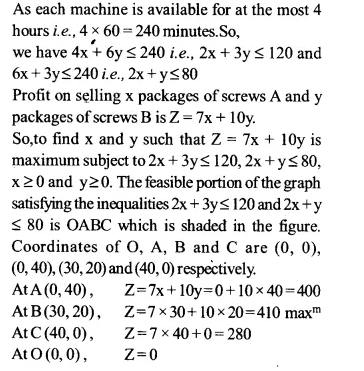Ex 12.2 Class 12 Maths Question 6.
A cottage industry manufactures pedestal lamps and wooden shades, each requiring the use of a grinding/ cutting machine and a sprayer. It takes 2 hours on grinding/ cutting machine and 3 hours on the sprayer to manufacture a pedestal lamp, while it takes 1 hour on the grinding/ cutting machine and 2 hours on the sprayer to manufacture a shade. On any day, the sprayer is available for at the most 20 hours and the grinding/cutting machine for at the most 12 hours. The profit from the sale of a lamp is Rs 5 and that from a shade is Rs 3. Assuming that the manufacturer can sell all the lamps and shades that he produces, how should he schedule his daily production in order to maximise his profit?
Solution:
Let the manufacturer produces x pedestal lamps and y wooden shades; then the time taken by x pedestal lamps and y wooden shades on grinding/ cutting machines = (2x + y) hours and time taken by x pedestal lamps and y shades on the sprayer = (3x + 2y) hours.
Since grinding/ cutting machine is available for at the most 12 hours, 2x + y ≤ 12 and sprayer is available for at the most 20 hours.
We have: 3x + 2y ≤ 20.
Profit from the sale of x lamps and y shades.
Z = 5x + 3y
So, our problem is to maximize Z = 5x + 3y subject to constraints 3x + 2y ≤ 20,2x + y ≤ 12, x, y ≥ 0.
Consider 3x + 2y ≤ 20
Let 3x + 2y = 20$y=frac { 20-3x }{ 2 }$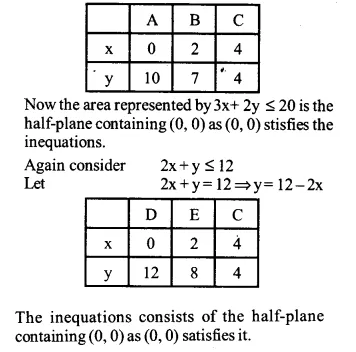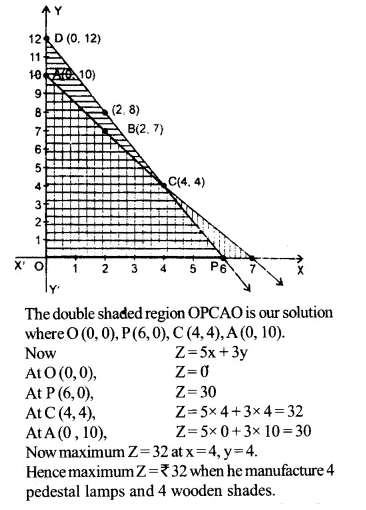Ex 12.2 Class 12 Maths Question 7.
A company manufactures two types of novelty souvenirs made of plywood. Souvenirs of type A require 5 minutes each for cutting and 10 minutes each for assembling. Souvenirs of type B require 8 minutes each for cutting and 8 minutes each for assembling. There are 3 hours 20 minutes available for cutting and 4 hours for assembling. The profit is Rs 5 each for type A and Rs 6 each for type B souvenirs. How many souvenirs of each type should be company manufacture in order to maximise the profit?
Solution:
Let the company manufactures x souvenirs of type A and y souvenirs of type B, then time taken for cutting x souvenirs of type A and y souvenirs of type A and y souvenirs of type B = (5x + 8y) minutes.
Since 3 hours 20 minutes i.e 200 minutes are available for cutting, so we should have 5x + 8y ≤ 200
Also as 4 hours i.e., 240 minutes are available for assembling, so we have 10x + 8y ≤ 240 i. e., 5x + 4y ≤ 120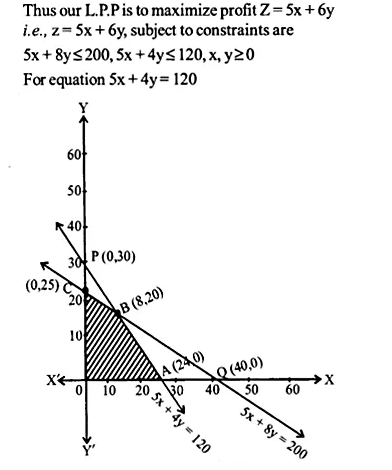Ex 12.2 Class 12 Maths Question 8.
A merchant plans to sell two types of personal computers – a desktop model and a portable model that will cost Rs 25000 and Rs 40000 respectively. He estimates that the total monthly demand of computers will not exceed 250 units. Determine the number of units of each type of computers which die merchant should stock to get maximum profit if he does not want to invest more than Rs 70 lakhs and if his profit on the desktop model is Rs 4500 and on portable model is Rs 5000.
Solution:
Let there be x desktop and y portable computers. Total monthly demand of computer does not exceed 250. => x+y ≤ 250, cost of 1 desktop computer if Rs 25000 and 1 portable computer is Rs 40000
∴ cost of x desktop and y portable computer = Rs (25000 x+40000 y)
Maximum investment = Rs 70 lakhs = Rs 70,00,000
=> 25000 x+40000 y≤ 7000000 or 5x+8y ≤ 1400
profit on 1 desktop computer is Rs 4500 and on 1 portable is Rs 5000, total profit,
Z = 4500 x+5000 y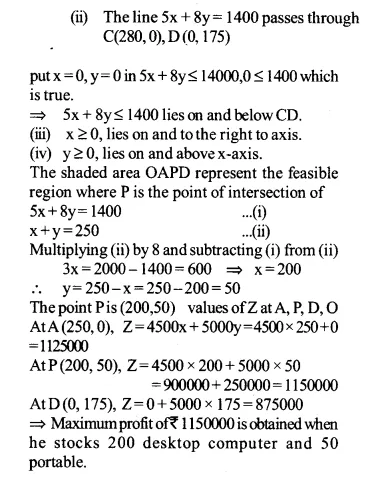Ex 12.2 Class 12 Maths Question 9.
A diet is to contain at least 80 units of vitamin A and 100 units of minerals. Two foods Ft and F2 are available. Food F1 costs Rs 4 per unit food and F2 costs Rs 6 per unit.One unit of food F2 contains 3 units of vitamin A and 4 units of minerals. One unit of food F2 contains 6 units of vitamin A and 3 units of minerals. Formulate this as a linear programming problem. Find the minimum cost for diet that consists of mixture of these two foods and also meets the minimal nutritional requirements.
Solution:
Let there be x units of food F1 and y units of food F2.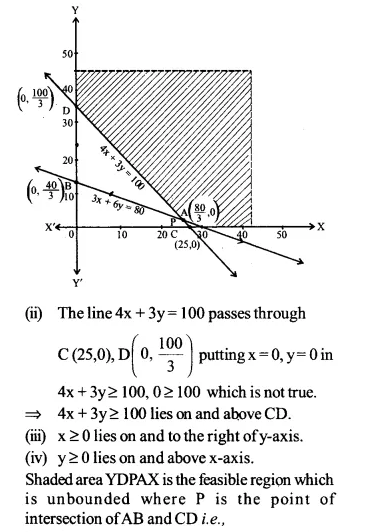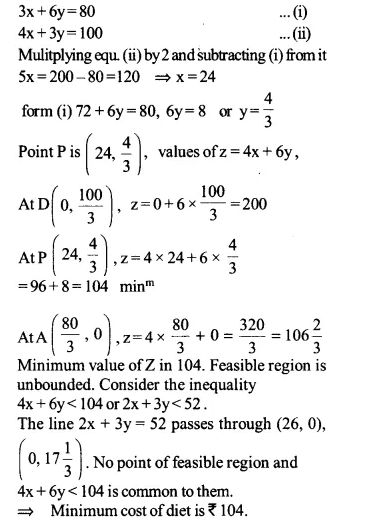Ex 12.2 Class 12 Maths Question 10.
There are two types of fertilisers F1, and F2. F1 consists of 10% nitrogen and 6% phosphoric acid and F2 consists of 5% nitrogen and 10% phosphoric acid. After testing the soil conditions, a fanner finds that she needs atleast 14 kg of nitrogen and 14 kg of phsophoric acid for her crop. If F2 costs Rs 6/kg and F2 costs Rs 5/ kg, determine how much of each type of fertiliser should be used so that nutrient requirements are met at a minimum cost What is the minimum cost?
Solution:
Let x kg of festiliser and y kg of fertilised F2. be required.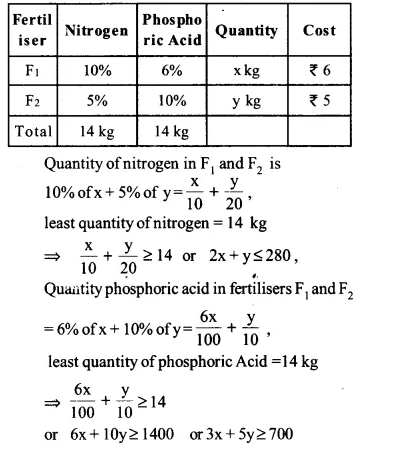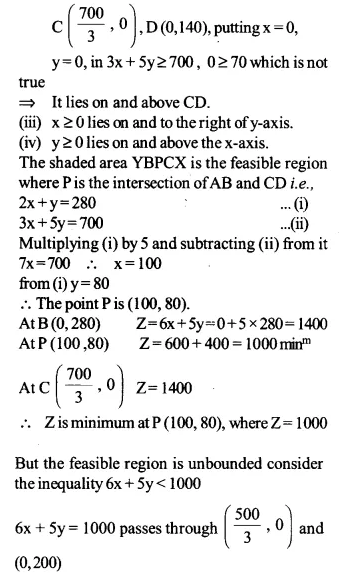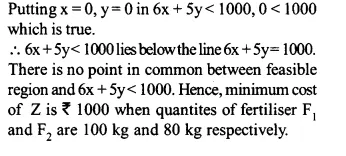Ex 12.2 Class 12 Maths Question 11.
The corner points of the feasible region determined by the following system of linear inequalities:
2x+y≤10,x+3y≤15, x,y≥0 are (0,0),(5,0), (3,4) and (0,5).
Let Z=px+qy, where p, q > 0. Condition on p and q so that the maximum of Z occurs at both (3,4) and (0,5) is
(a) p=q
(b) p=2q
(c) p=3q
(d) q=3p
Solution:
Maximum value of Z = px + qy occurs at (3,4) and (0,5),
At (3,4), Z = px + qy = 3p + 4q
At (0,5), Z = 0 + q . 5 = 5q
Both are the maximum values
=> 3p + 4q = 5q or q = 3p
Option (d) is correct.